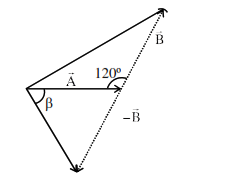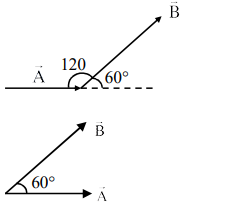# Solve this following

Question:

The angle between vector $(\overrightarrow{\mathrm{A}})$ and $(\overrightarrow{\mathrm{A}}-\overrightarrow{\mathrm{B}})$ is :1. $\tan ^{-1}\left(\frac{-\frac{B}{2}}{A-B \frac{\sqrt{3}}{2}}\right)$

2. $\tan ^{-1}\left(\frac{A}{0.7 B}\right)$

3. $\tan ^{-1}\left(\frac{\sqrt{3} B}{2 A-B}\right)$

4. $\tan ^{-1}\left(\frac{\mathrm{B} \cos \theta}{\mathrm{A}-\mathrm{B} \sin \theta}\right)$

Correct Option: , 3

Solution:Angle between $\overrightarrow{\mathrm{A}}$ and $\overrightarrow{\mathrm{B}}, \theta=60^{\circ}$

Angle betwenn $\overrightarrow{\mathrm{A}}$ and $\overrightarrow{\mathrm{A}}-\overrightarrow{\mathrm{B}}$

$\tan \alpha=\frac{B \sin \theta}{A-B \cos \theta}$

$=\frac{B \sqrt{\frac{3}{2}}}{A-B \times \frac{1}{2} 2}$

$\tan \alpha=\frac{\sqrt{3} B}{2 A-B}$

Ans 3